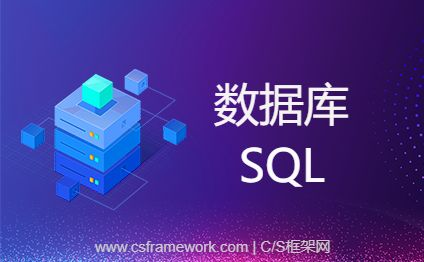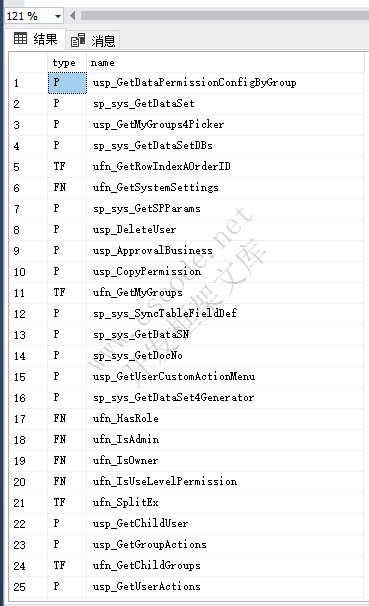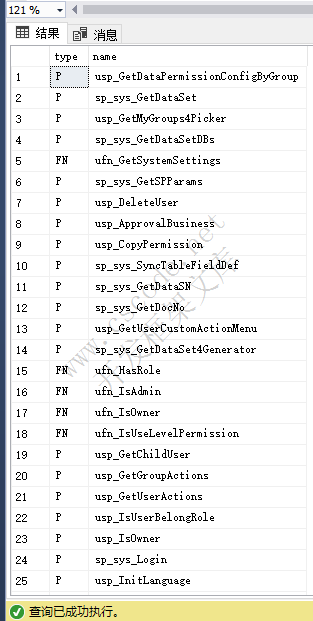# SQL获取数据库中所有用户自定义存储过程、函数、触发器SQL获取数据库中所有用户自定义存储过程、函数、触发器

## 获取存储过程、函数、触发器

SQL 全选
``select type,name  from sysobjects where xtype in ('p','fn','tr') and category=0``## 获取存储过程和函数

SQL 全选
``select type,name  from sysobjects where xtype in ('p','fn') and category=0``## xtype 类型说明

xtype char(2) 对象类型。可以是下列对象类型中的一种：

AF = 聚合函数 (CLR)

C = CHECK 约束

D = 默认值或 DEFAULT 约束

F = FOREIGN KEY 约束

L = 日志

FN = 标量函数

FS = Assembly (CLR) 标量函数

FT = Assembly (CLR) 表值函数

IF = 内嵌表函数

P = 存储过程

PC = Assembly (CLR) 存储过程

PK = PRIMARY KEY 约束（类型是 K）

RF = 复制筛选存储过程

S = 系统表

TF = 表函数

TR = 触发器

U = 用户表

UQ = UNIQUE 约束（类型是 K）

V = 视图

X = 扩展存储过程

AF = Aggregate function (CLR)

C = CHECK constraint

D = Default or DEFAULT constraint

F = FOREIGN KEY constraint

L = Log

FN = Scalar function

FS = Assembly (CLR) scalar-function

FT = Assembly (CLR) table-valued function

IF = In-lined table-function

IT = Internal table

P = Stored procedure

PC = Assembly (CLR) stored-procedure

PK = PRIMARY KEY constraint (type is K)

RF = Replication filter stored procedure

S = System table

SN = Synonym

SQ = Service queue

TA = Assembly (CLR) DML trigger

TF = Table function

TR = SQL DML Trigger

TT = Table type

U = User table

UQ = UNIQUE constraint (type is K)

V = View

X = Extended stored procedureC/S框架网站长推荐